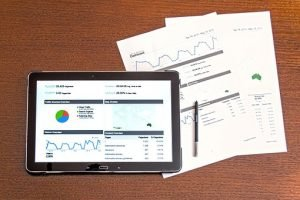# How to Code and Enter Data in SPSSData analysis involves running raw data in a statistical program to obtain results. Analysis can only be done after you code and enter data in SPSS.

Statistical methods such as descriptive statistics and inferential statistics aid a researcher understand data patterns. Normally statisticians use SPSS, SaS, STATA, S-Plus, R GUI and MS EXCEL to conduct statistical and mathematical analysis. The selection of a package depends on familiarity and simplicity of a program to the user.

SPSS stands for Statistical Package for Social Scientists; the package is mostly used by non-statisticians due to its simplicity. It has a graphical user interface that makes it friendly to users with little programming language. This is unlike other packages like R and SaS that are command oriented and require users to be conversant with statistical equations modeling. Before running analysis using SPSS a user need learn how to code and enter data in SPSS system

In SPSS, the first step involves defining the names and inherent traits of the variable. This is followed by entering values into each defined variable. Each row and column represents source of data and characteristics of the measured data respectively. In case, you wish to add variables after entering data, you just need to define new variables in the variable view. Go to variable view, click an empty row and start defining variables as stated below.

## Code and Enter Data in SPSS Like an ExpertBefore you run an analysis in SPSS, you will be required to code and enter data in SPSS. The process is so simple that you can do it within 10 minutes even for large data-sets.The process of coding data is described below:

Go to variable view, click an empty row and start defining variables as stated below.

• Name: SPSS requires that each variable to be unique, and contain a maximum of 8 characters.
• Type: There are eight types of variables to be found in SPSS that include numeric, comma, dot, scientific notation, date, dollar, custom curency and string. To select variable type, click in the cell on the grey box.
• Width: This refers to the number of characters to be inputted for the variable.
• Decimals: This is the number of decimal places to be displayed by the program
• Label: This is a string of text that explains in details what a variable represents. You can enter a maximum of 255 characters containing spaces and punctuation marks.
• Values:  In case of categorical data, you need to specify which numbers represent which category. For example when coding gender in SPSS you can let 1 represent male and 2 represent female. This can be seen in the data view by clicking the toe tag icon. On clicking the tag it switched between numeric values and their labels.
• Missing: This entry in the variable view signals to the program that data is missing. You need to assign figures to be missing values. Like by assigning 9,99 and 999 as discrete missing vales. SPSS would treat these figures as missing and ignore the values.
• Measure: This property indicates level of measurement of data source. The three measure properties include scale, ordinal and nominal. Interval and ratio levels of measurement are grouped as scale measures.

Once you are done with coding data, you can enter data values in the data view screen. This will be followed by data analysis depending on what you wish to achieve.

Code and Enter Data in SPSS To Begin Statistical AnalysisThere are two types of analysis namely descriptive and inferential analysis. Descriptive analysis seeks to study frequency and features exhibited by the data.

The most common descriptive statistical methods include measure of central tendency and measure of dispersion. These statistical measures are presented in the form of frequency tables, pie charts, histograms and boxplots. These tables and charts summarize data and scale variables.

Inferential analysis goes further to study how variables in a data set relate with each other and forecasting outcome. Survival analysis, modeling, regression, factor analysis, discriminant analysis, time series and categorical tests are some of the inferential statistical methods. Besides researchers, inferential statistical analysis are widely being used by data scientists in studying data patterns and predicting future outcomes.

In case you are any problems, get in expert data analysis help in SPSS from our SPSS experts online.